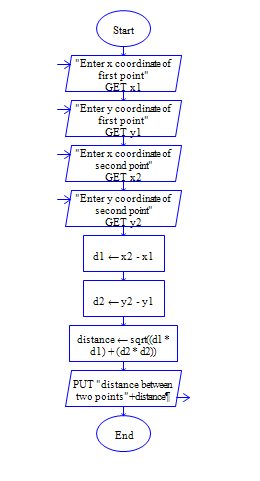# Java Program to find the distance between two points in 2D space

Get x and y coordinates of two points(x1,y1,x2,y2) and find distance between two points

Sample Input 1:

3 4 4 5

Sample Output 1:

1.414

#### Flow Chart Design#### Program or Solution

``` import java.util.*; import java.lang.Math.*; class Distance { public static void main(String args[]) { int x1,x2,y1,y2; int x,y; double distance; Scanner sc=new Scanner(System.in); System.out.println("Enter The Coordinates:"); x1=sc.nextInt(); x2=sc.nextInt(); y1=sc.nextInt(); y2=sc.nextInt(); x=x2-x1; y=y2-y1; distance=Math.sqrt((x*x)+(y*y)); System.out.println("The Distance Is:"+distance ); } } ```

#### Program Explanation

Get x1,y1,x2 and y2 coordinates of two points(using scanner class) Calculate distance by the eculdian distance formula. Math.sqrt is function available in java library to find square root. Print distance (using system.out.println).